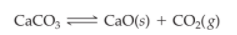×
Get Full Access to Introductory Chemistry - 5 Edition - Chapter 15 - Problem 103p
Get Full Access to Introductory Chemistry - 5 Edition - Chapter 15 - Problem 103p

×

# Consider the reaction: A sample of CaCO3 is placed into a sealed 0.500-L container andISBN: 9780321910295 34

## Solution for problem 103P Chapter 15

Introductory Chemistry | 5th Edition

• Textbook Solutions
• 2901 Step-by-step solutions solved by professors and subject experts
• Get 24/7 help from StudySoup virtual teaching assistantsIntroductory Chemistry | 5th Edition

4 5 1 261 Reviews
11
3
Problem 103P

Consider the reaction:

$$\mathrm{CaCO}_{3} \rightleftharpoons \mathrm{CaO}(s)+\mathrm{CO}_{2}(g)$$

A sample of $$\mathrm{CaCO}_{3}$$ is placed into a sealed 0.500-L container and heated to 550 K at which the equilibrium constant is $$4.1^{*} 10^{-4}$$. When the reaction has come to equilibrium, what mass of solid CaO is in the container? (Assume that the sample of $$\mathrm{CaCO}_{3}$$ was large enough

that equilibrium could be achieved.)

Equation Transcription:CaCO3

4.1 * 10-4

Text Transcription:

CaCO_3 Harpoon CaO(s) + CO_2(g)

CaCO_3

4.1 times 10^-4

Step-by-Step Solution:

Solution:

Step 1The equilibrium constant is

Keq =It is known that the equilibrium constants for pure solids and liquids are zero. So that [CaCO3(s)] and [CaO(s)] will be equal to zero. The above equation can be written as,

Keq = [CO2]

Here it has been given that Keq = 4.1 × 10−4

Thus Keq = [CO2] = 4.1× 10−4 = 0.00041M

The mole of CaO = 0.5 L× 0.00041 mol/L= 0.000205 mol

Molar mass of CaO = 56.07g/mol

Thus mass of CaO in the container = 56.07 g/mol  × 0.000205 mol = 0.0115g

Thus the container have 0.0115 g of CaO.

Step 2 of 1

## Discover and learn what students are asking

Calculus: Early Transcendental Functions : Area and Arc Length in Polar Coordinates
?In Exercises 5-16, find the area of the region. One petal of $$r=2 \cos 3 \theta$$

Calculus: Early Transcendental Functions : Conservative Vector Fields and Independence of Path
?In Exercises 1 - 4, show that the value of $$\int_{C} F \cdot d r$$ is the same for each parametric representation of C. \(\mathbf{F}(x, y)=\

Statistics: Informed Decisions Using Data : Testing the Significance of the Least-Squares Regression Model
?Why don’t we conduct inference on the linear correlation coefficient?

Unlock Textbook Solution